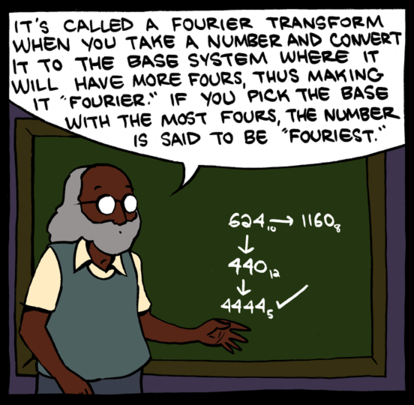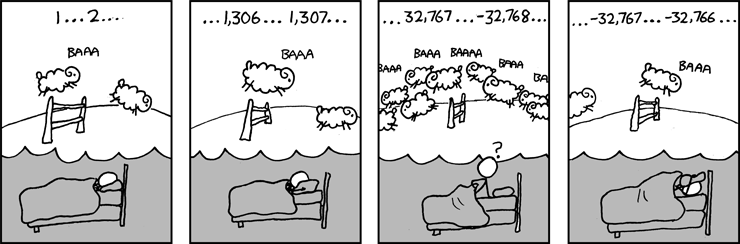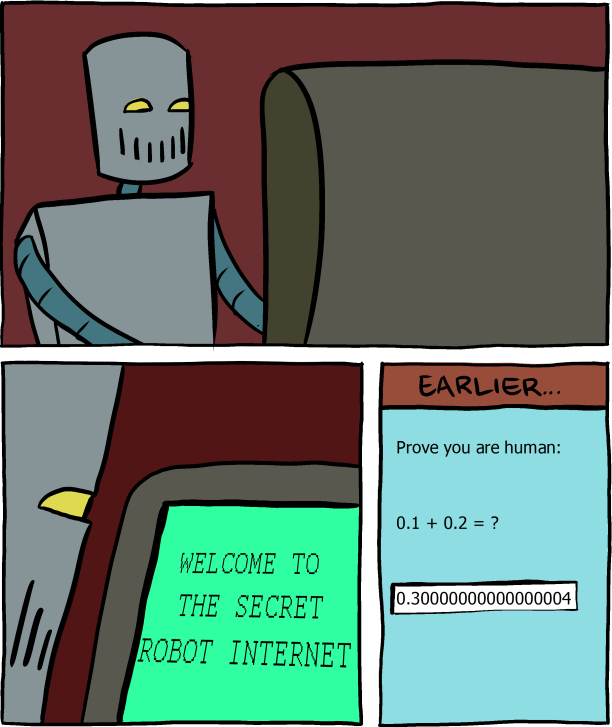# Number systems and binaryIn the first unit of this course, we look at binary numbers and binary representations of other sorts of data.

## 1 Positional numbering system

Our normal number system is a positional system, where the position (column) of a digit represents its value. Starting from the right, we have the ones column, tens column, hundreds, thousands, and so on. Thus the number 3724 stands for three THOUSAND, seven HUNDRED, two TENS (called twenty), and four ONES.

The values of those columns derive from the powers of ten, which is then called the base of the number system. The base ten number system is also called decimal.

There is nothing special about base ten, except that it’s what you learned from a young age. A positional numbering system can use any quantity as its base. Let’s take, for example, base five. In base five, the columns represent the quantities (from right to left) one, five, twenty-five, and a hundred twenty-five. We need to use five symbols to indicate quantities from zero up to four. For simplicity, let’s keep the same numerals we know: 0, 1, 2, 3, and 4.

The number shown in this figure (3104) represents the same quantity that we usually write as 404 in base ten. That’s because it is three “hundred twenty-fives” (= 375), plus one “twenty-five” (= 25) plus four ones (= 4), so 375 + 25 + 4 = 404.

You can count directly in base five; it looks like this: 0, 1, 2, 3, 4, 10, 11, 12, 13, 14, 20, 21, 22, 23, 24, 30, 31, 32, 33, 34, 40, 41, 42, 43, 44, 100. (Those correspond to quantities from zero to twenty-five.)

Here is an algorithm for converting from base ten to any foreign base. Suppose we have the number 344 (three hundred forty-four) that we’d like to write in base five.

We start by dividing the number by the desired base, so 344 ÷ 5 = 68.8. It helps to think of that as 68 with a remainder of 4. (The .8 corresponds to a remainder of 4 because .8 × 5 = 4.) Remember the remainder, but proceed with the whole-number part. So now we divide 68 ÷ 5 = 13.6 which is remainder 3. Next, 13 ÷ 5 = 2.6, again remainder 3. Finally, 2 ÷ 5 = 0.4, which is remainder 2.

We stop when the whole-number part becomes zero, and then write the remainders from right to left: 2334. Thus, 344 in base ten is written as “2334” in base 5.

### Further explorationFigure 3: @wirehead2501 on TwitterFigure 4: Teaching math was way more fun after tenure — from Saturday Morning Breakfast Cereal

## 2 Binary numbers

Computer systems use binary numbers – that just means they are expressed in base two. Using two as the base is really convenient and flexible, because we need only two symbols and there are so many ways we can represent them: zero/one, on/off, up/down, high/low, positive/negative, etc.

In binary, the columns are (from right to left) 1, 2, 4, 8, 16, 32, and so on. Using a zero means we exclude that column’s quantity, and a one means we include it.

So the binary number 10110 is the quantity 16 + 4 + 2 = 22. Each binary digit (a one or a zero) is called a bit. The largest five-bit binary number, then is 11111 = 16 + 8 + 4 + 2 + 1 = 31.

It’s worthwhile to learn to count in binary, at least from zero to fifteen:

```0000 = 0    0100 = 4    1000 =  8    1100 = 12
0001 = 1    0101 = 5    1001 =  9    1101 = 13
0010 = 2    0110 = 6    1010 = 10    1110 = 14
0011 = 3    0111 = 7    1011 = 11    1111 = 15
```

The repeated division algorithm we learned in the positional numbering section also works for binary, but there’s an even simpler way it can be adapted, by thinking in terms of even and odd numbers.

Let’s convert the number 46 to binary. We begin by noticing that it is even, so we write a zero. Then we divide the number in half to get 23. That number is odd, so we write a one. Then we divide it in half (discarding the .5 remainder) to get 11. That’s odd, so we write a one, and so on.

```46  even  0
23  odd   1
11  odd   1
5  odd   1
2  even  0
1  odd   1
```

The algorithm ends when we get down to 1, and then we read the binary number from bottom to top: 101110 is the binary representation of the quantity 46.

## 3 Binary arithmetic

It’s relatively easy to add numbers directly in binary. Line up the columns and then proceed from right to left, as usual. There are only four possible cases:

• If a column has no ones, write a zero below.
• If a column has one one, write a one below.
• If a column has two ones, write a zero and carry a one to the next column (to the left).
• Finally, if a column has three ones (possible due to an incoming carry), write a one and carry a one to the next column.

Below is an example of adding 10110 plus 11100. The result is 110010, and you can see the carry bits above the original numbers, in orange.

When adding this way, it’s always a good idea to check your work by converting the numbers to decimal and checking the addition. In this case, we’re adding 22 (10110) to 28 (11100) to get 50 (110010).

## 4 Fixed-size binary numbers

We generally arrange numbers along a line that continues into infinity in both directions. In binary we can always continue counting by adding more and more columns that are powers of two.

However, in most computer systems and programs we use fixed-size numbers. That is, we decide in advance how many bits will be used to represent the number. For example, a 32-bit computer represents most of its numbers and addresses using 32 bits. The largest such number is 232 − 1 = 4,294,967,295. Many computers now use 64 bits. The largest 64-bit number is 264 − 1 = 18,446,744,073,709,551,615.

When your numbers have a fixed size, then there is no number line heading off into infinity. Instead, we arrange the numbers around a circle, like a clock. Below is the number wheel for 3-bit integers. The smallest 3-bit integer is zero, and the largest is seven. Then, if you attempt to keep counting, it just wraps around to zero again.

When you perform arithmetic with fixed-size numbers, you throw away any extra carry bit; the result cannot exceed the designated size. For example, see what happens if we try to add 110 + 011 using 3-bit integers:

In 3-bit arithmetic, 6 plus 3 is 1. You can make sense of this on the number wheel. Addition corresponds to walking clock-wise around the wheel. So start at 6, and go clockwise by 3. That lands on 1, which is 6+3.

## 5 Signed magnitude

Now we’ll look at signed numbers – that is, numbers that can be positive or negative. There are two techniques for encoding signed numbers. The first one is called signed magnitude. It appears simple at first, but that simplicity hides some awkward properties.

Here’s how it works. We use a fixed width, and then the left-most bit represents the sign. So 4-bit signed magnitude looks like this:

```
————  ————  ————  ————
sign    4     2     1
```

where having the sign bit set to `1` means the magnitude is interpreted as negative. Thus, `0110` is `+6` whereas `1110` is `-6`. In this system, the largest positive number is `0111 = +7` and the most negative number is `1111 = -7`.

One of the unfortunate effects of this representation is there are two ways to write zero: `0000` and also `1000`. There is no such thing as negative zero, so this doesn’t really make sense.

## 6 Two’s complement

The second way to represent signed quantities is called two’s complement. Although this looks trickier at first, it actually works really well. Below is the interpretation of 4-bit two’s complement. All we need to do compared to normal unsigned numbers is negate the value of the left-most bit.

```  1     0     1     0   =  -6
————  ————  ————  ————
-8     4     2     1
```

So `+6` is `0110` as before, but what about `-6`? We need to turn on the negative 8, and then add two: `1010`. To represent `-1`, you turn on all the bits: `1111`, because that produces `-8+4+2+1 = -8+7 = -1`.

The nice thing about two’s complement is that you can add these numbers and everything just works out. Let’s try adding `7` and `-3`:

``` 0 1 1 1  =   7
1 1 0 1  =  -3
—————————   ————
0 1 0 0  =   4
```

It’s also relatively easy to negate a number – that is, to go from `+6` to `-6` or from `-3` to `+3`. Here are the steps:

1. First, flip all the bits. That is, all the zeroes become ones and all the ones become zeroes.
2. Next, add one.

For example here is how we produce `-6` from `+6`:

``` 0 1 1 0  =  +6
—————————
1 0 0 1  (flip all the bits)
+ 1  (add one)
—————————
1 0 1 0  =  -6
```

You don’t even have to reverse these steps in order to convert back:

``` 1 0 1 0  =  -6
—————————
0 1 0 1  (flip all the bits)
+ 1  (add one)
—————————
0 1 1 0  =  +6
```Figure 9: “Can’t Sleep” from XKCD. How many bits is this person using?

## 7 Octal and hexadecimal

Finally, I want to introduce two number systems that are very useful as abbreviations for binary. They work so well because their bases are powers of two.

Octal is base eight, so we use the symbols 0–7 and the values of the columns are:

```
———  ———  ———  ———
512   64   8    1
8³   8²   8¹   8⁰
```

The real advantage of octal, however, is that each octal digit maps to exactly three binary digits. So, an octal number like `3714` maps as shown:

```   3       7       1       4     octal number
——————— ——————— ——————— ———————
0 1 1   1 1 1   0 0 1   1 0 0   binary number
(4 2 1) (4 2 1) (4 2 1) (4 2 1)
```

Both of those numbers are equal to `1996` in base ten, but it’s more difficult to convert to base ten than between octal and binary.Figure 10: The DEC PDP-8 (1970) was programmed partly using octal, so its switches and lights were grouped into threes. [Photo: dustyoldcomputers.com]

Hexadecimal is base sixteen, so we use the symbols 0–9 and then `A` to represent ten, `B` for eleven, `C` for twelve, and so on up to `F` for fifteen. The values of the columns are:

```
————  ————  ————  ————
4096   256   16     1
16³   16²   16¹   16⁰
```

So a hexadecimal number like `2A5C` has the value `2×4096 + 10×256 + 5×16 + 12×1 = 10844` in base ten.

In hexadecimal, each digit maps to exactly four bits. So here is that same number in binary:

```    2         A         5         C
————————— ————————— ————————— —————————
0 0 1 0   1 0 1 0   0 1 0 1   1 1 0 0
(8 4 2 1) (8 4 2 1) (8 4 2 1) (8 4 2 1)
```

### Further exploration

Below are two great video explanations of hexadecimal. (Note when you watch these – the British presenters pronounce zero as “naught.”)

## 8 Practice problems

You can reveal solutions by tapping on a blank answer. Tap again to hide. Or you can download:
PDF with solutions
PDF without solutions

1. Convert the base ten (decimal) number `83` into the following bases:
• base 4 : 1103
• base 5 : 313
• base 6 : 215
• base 7 : 146
2. Convert the following numbers from the specified bases into base ten.
• `232`4 = 46
• `414`5 = 109
• `205`6 = 77
• `164`7 = 95
3. Convert the following base ten (decimal) numbers into binary.
• `  6`  =     110
• ` 18`  =   10010
• ` 51`  =  110011
• ` 63`  =  111111
4. Convert the following unsigned binary numbers into base ten.
• `  1010`  =  10 (ten)
• `  1101`  =  13
• `  1000`  =  8
• ` 10001`  =  17
5. What do all odd numbers have in common, when written in binary? (Hint: try writing the quantities 3, 5, 7, 9 in binary.)

Show answers

6. Using 7-bit signed two’s complement binary numbers, what is the largest positive number? What is the most negative number?

Show answers

7. Convert the following 5-bit signed two’s complement binary numbers into base ten.
• `01101`  =  +13
• `01111`  =  +15
• `10011`  =  -13
• `11111`  =  -1
8. Convert the following 16-bit binary number into octal and hexadecimal.
```0 1 1 1 1 1 1 1 0 0 1 1 1 0 1 0
```

Show answers

9. Convert the following hexadecimal numbers into binary:
• `  9D`  =  1001,1101
• `  C4`  =  1100,0100
• ` D05`  =  1101,0000,0101
• `A17E`  =  1010,0001,0111,1110
10. Convert the following octal numbers into binary:
• `  37`  =  11,111
• ` 415`  =  100,001,101
• ` 620`  =  110,010,000
11. Add and verify the following unsigned binary numbers.
```  1 0 1 1 1 1
+   1 1 1 0 1
——————————————
```

Show answers

## 9 Extra: floating-pointFigure 11: jaffathecake on TwitterFigure 12: CAPTCHA for the Secret Robot Internet — from Saturday Morning Breakfast Cereal